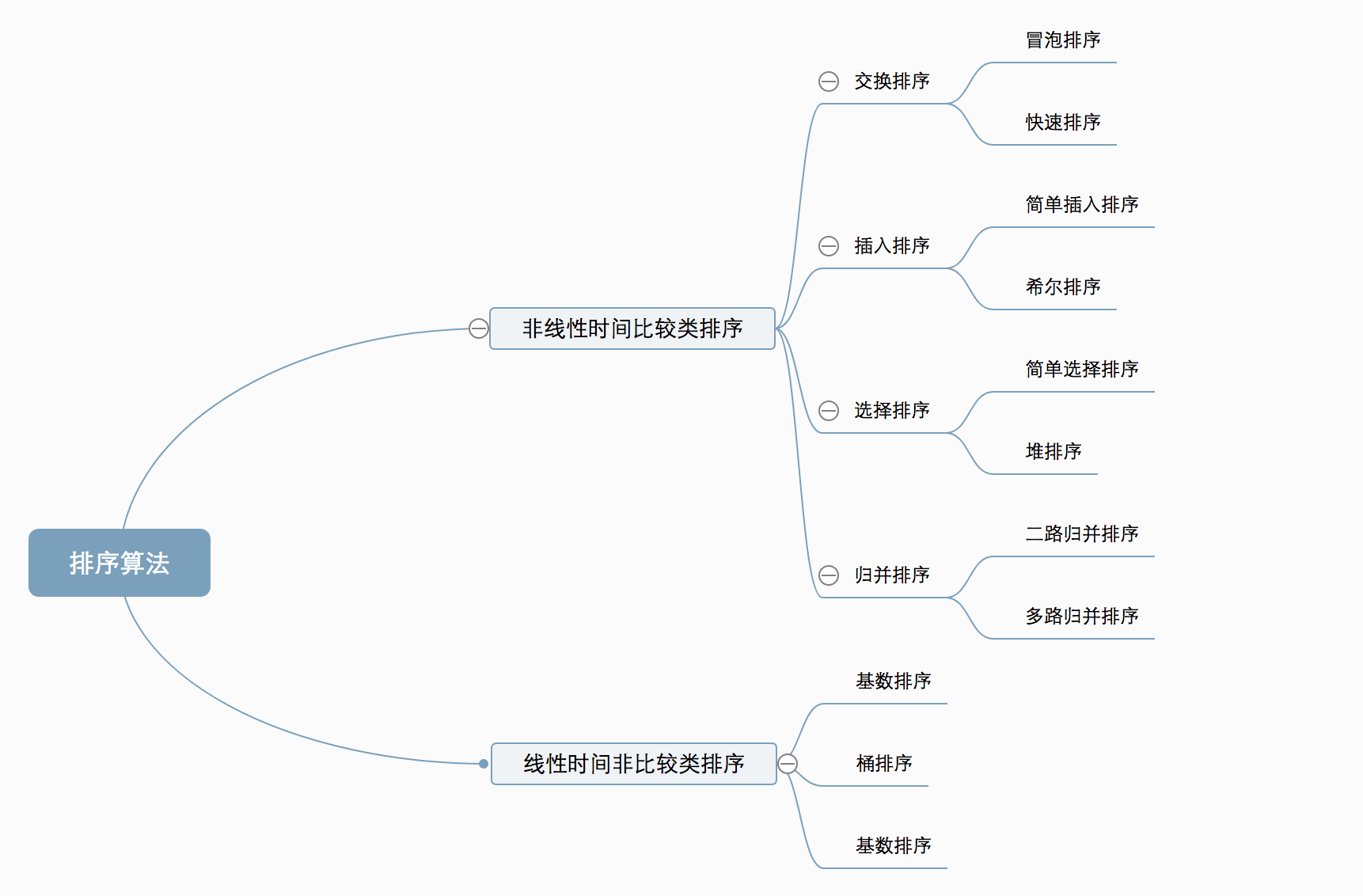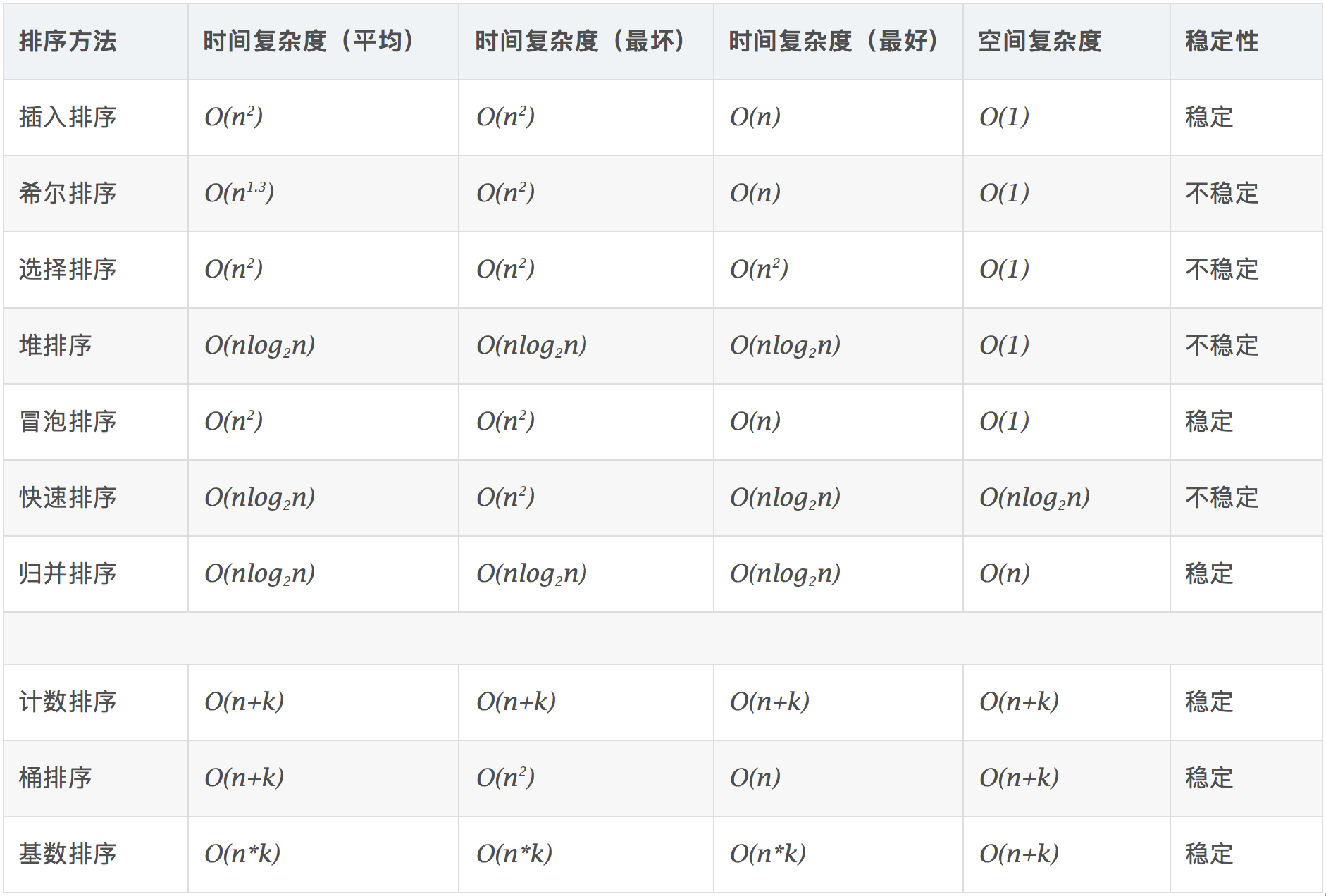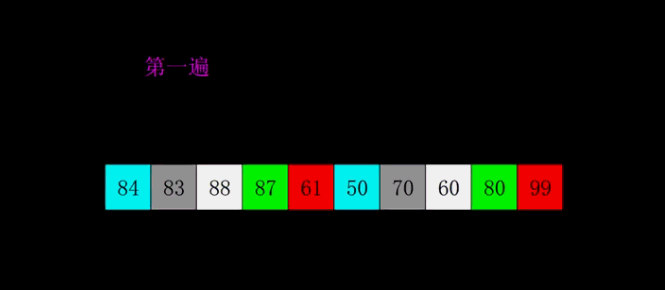# 基础算法之一：排序算法

### 0、算法概述

0.1 算法分类0.2 算法复杂度0.3 相关概念

### 1、冒泡排序（Bubble Sort）

1.1 算法描述

• 比较相邻的元素。如果第一个比第二个大，就交换它们两个；
• 对每一对相邻元素作同样的工作，从开始第一对到结尾的最后一对，这样在最后的元素应该会是最大的数；
• 针对所有的元素重复以上的步骤，除了最后一个；
• 重复步骤1~3，直到排序完成。

1.2 动图演示1.3 代码实现

 1 2 3 4 5 6 7 8 9 10 11 12 13 function bubbleSort(arr) {     var len = arr.length;     for (var i = 0; i < len - 1; i++) {         for (var j = 0; j < len - 1 - i; j++) {             if (arr[j] > arr[j+1]) {       // 相邻元素两两对比                 var temp = arr[j+1];       // 元素交换                 arr[j+1] = arr[j];                 arr[j] = temp;             }         }     }     return arr; }

### 2、选择排序（Selection Sort）

2.1 算法描述

n个记录的直接选择排序可经过n-1趟直接选择排序得到有序结果。具体算法描述如下：

• 初始状态：无序区为R[1..n]，有序区为空；
• 第i趟排序(i=1,2,3…n-1)开始时，当前有序区和无序区分别为R[1..i-1]和R(i..n）。该趟排序从当前无序区中-选出关键字最小的记录 R[k]，将它与无序区的第1个记录R交换，使R[1..i]和R[i+1..n)分别变为记录个数增加1个的新有序区和记录个数减少1个的新无序区；
• n-1趟结束，数组有序化了。

2.2 动图演示2.3 代码实现

 1 2 3 4 5 6 7 8 9 10 11 12 13 14 15 16 function selectionSort(arr) {     var len = arr.length;     var minIndex, temp;     for (var i = 0; i < len - 1; i++) {         minIndex = i;         for (var j = i + 1; j < len; j++) {             if (arr[j] < arr[minIndex]) {    // 寻找最小的数                 minIndex = j;                // 将最小数的索引保存             }         }         temp = arr[i];         arr[i] = arr[minIndex];         arr[minIndex] = temp;     }     return arr; }

2.4 算法分析

### 3、插入排序（Insertion Sort）

3.1 算法描述

• 从第一个元素开始，该元素可以认为已经被排序；
• 取出下一个元素，在已经排序的元素序列中从后向前扫描；
• 如果该元素（已排序）大于新元素，将该元素移到下一位置；
• 重复步骤3，直到找到已排序的元素小于或者等于新元素的位置；
• 将新元素插入到该位置后；
• 重复步骤2~5。

3.2 动图演示3.2 代码实现

 1 2 3 4 5 6 7 8 9 10 11 12 13 14 function insertionSort(arr) {     var len = arr.length;     var preIndex, current;     for (var i = 1; i < len; i++) {         preIndex = i - 1;         current = arr[i];         while (preIndex >= 0 && arr[preIndex] > current) {             arr[preIndex + 1] = arr[preIndex];             preIndex--;         }         arr[preIndex + 1] = current;     }     return arr; }

3.4 算法分析

### 4、希尔排序（Shell Sort）

1959年Shell发明，第一个突破O(n2)的排序算法，是简单插入排序的改进版。它与插入排序的不同之处在于，它会优先比较距离较远的元素。希尔排序又叫缩小增量排序

4.1 算法描述

• 选择一个增量序列t1，t2，…，tk，其中ti>tj，tk=1；
• 按增量序列个数k，对序列进行k 趟排序；
• 每趟排序，根据对应的增量ti，将待排序列分割成若干长度为m 的子序列，分别对各子表进行直接插入排序。仅增量因子为1 时，整个序列作为一个表来处理，表长度即为整个序列的长度。

4.2 动图演示4.3 代码实现

 1 2 3 4 5 6 7 8 9 10 11 12 13 14 15 16 17 18 function shellSort(arr) {     var len = arr.length,         temp,         gap = 1;     while (gap < len / 3) {         // 动态定义间隔序列         gap = gap * 3 + 1;     }     for (gap; gap > 0; gap = Math.floor(gap / 3)) {         for (var i = gap; i < len; i++) {             temp = arr[i];             for (var j = i-gap; j > 0 && arr[j]> temp; j-=gap) {                 arr[j + gap] = arr[j];             }             arr[j + gap] = temp;         }     }     return arr; }

4.4 算法分析

### 5、归并排序（Merge Sort）

5.1 算法描述

• 把长度为n的输入序列分成两个长度为n/2的子序列；
• 对这两个子序列分别采用归并排序；
• 将两个排序好的子序列合并成一个最终的排序序列。

5.2 动图演示5.3 代码实现

 1 2 3 4 5 6 7 8 9 10 11 12 13 14 15 16 17 18 19 20 21 22 23 24 25 26 27 28 29 30 function mergeSort(arr) { // 采用自上而下的递归方法     var len = arr.length;     if (len < 2) {         return arr;     }     var middle = Math.floor(len / 2),         left = arr.slice(0, middle),         right = arr.slice(middle);     return merge(mergeSort(left), mergeSort(right)); }   function merge(left, right) {     var result = [];       while (left.length>0 && right.length>0) {         if (left <= right) {             result.push(left.shift());         }else {             result.push(right.shift());         }     }       while (left.length)         result.push(left.shift());       while (right.length)         result.push(right.shift());       return result; }

5.4 算法分析

### 6、快速排序（Quick Sort）

6.1 算法描述

• 从数列中挑出一个元素，称为 “基准”（pivot）；
• 重新排序数列，所有元素比基准值小的摆放在基准前面，所有元素比基准值大的摆在基准的后面（相同的数可以到任一边）。在这个分区退出之后，该基准就处于数列的中间位置。这个称为分区（partition）操作；
• 递归地（recursive）把小于基准值元素的子数列和大于基准值元素的子数列排序。

6.2 动图演示6.3 代码实现

 1 2 3 4 5 6 7 8 9 10 11 12 13 14 15 16 17 18 19 20 21 22 23 24 25 26 27 28 29 30 31 32 function quickSort(arr, left, right) {     var len = arr.length,         partitionIndex,         left =typeof left !='number' ? 0 : left,         right =typeof right !='number' ? len - 1 : right;       if (left < right) {         partitionIndex = partition(arr, left, right);         quickSort(arr, left, partitionIndex-1);         quickSort(arr, partitionIndex+1, right);     }     return arr; }   function partition(arr, left ,right) {    // 分区操作     var pivot = left,                     // 设定基准值（pivot）         index = pivot + 1;     for (var i = index; i <= right; i++) {         if (arr[i] < arr[pivot]) {             swap(arr, i, index);             index++;         }            }     swap(arr, pivot, index - 1);     return index-1; }   function swap(arr, i, j) {     var temp = arr[i];     arr[i] = arr[j];     arr[j] = temp; }

### 7、堆排序（Heap Sort）

7.1 算法描述

• 将初始待排序关键字序列(R1,R2….Rn)构建成大顶堆，此堆为初始的无序区；
• 将堆顶元素R与最后一个元素R[n]交换，此时得到新的无序区(R1,R2,……Rn-1)和新的有序区(Rn),且满足R[1,2…n-1]<=R[n]；
• 由于交换后新的堆顶R可能违反堆的性质，因此需要对当前无序区(R1,R2,……Rn-1)调整为新堆，然后再次将R与无序区最后一个元素交换，得到新的无序区(R1,R2….Rn-2)和新的有序区(Rn-1,Rn)。不断重复此过程直到有序区的元素个数为n-1，则整个排序过程完成。

7.2 动图演示7.3 代码实现

 1 2 3 4 5 6 7 8 9 10 11 12 13 14 15 16 17 18 19 20 21 22 23 24 25 26 27 28 29 30 31 32 33 34 35 36 37 38 39 40 41 42 43 44 var len;   // 因为声明的多个函数都需要数据长度，所以把len设置成为全局变量   function buildMaxHeap(arr) {  // 建立大顶堆     len = arr.length;     for (var i = Math.floor(len/2); i >= 0; i--) {         heapify(arr, i);     } }   function heapify(arr, i) {    // 堆调整     var left = 2 * i + 1,         right = 2 * i + 2,         largest = i;       if (left < len && arr[left] > arr[largest]) {         largest = left;     }       if (right < len && arr[right] > arr[largest]) {         largest = right;     }       if (largest != i) {         swap(arr, i, largest);         heapify(arr, largest);     } }   function swap(arr, i, j) {     var temp = arr[i];     arr[i] = arr[j];     arr[j] = temp; }   function heapSort(arr) {     buildMaxHeap(arr);       for (var i = arr.length - 1; i > 0; i--) {         swap(arr, 0, i);         len--;         heapify(arr, 0);     }     return arr; }

### 8、计数排序（Counting Sort）

8.1 算法描述

• 找出待排序的数组中最大和最小的元素；
• 统计数组中每个值为i的元素出现的次数，存入数组C的第i项；
• 对所有的计数累加（从C中的第一个元素开始，每一项和前一项相加）；
• 反向填充目标数组：将每个元素i放在新数组的第C(i)项，每放一个元素就将C(i)减去1。

8.2 动图演示8.3 代码实现

 1 2 3 4 5 6 7 8 9 10 11 12 13 14 15 16 17 18 19 20 21 22 function countingSort(arr, maxValue) {     var bucket =new Array(maxValue + 1),         sortedIndex = 0;         arrLen = arr.length,         bucketLen = maxValue + 1;       for (var i = 0; i < arrLen; i++) {         if (!bucket[arr[i]]) {             bucket[arr[i]] = 0;         }         bucket[arr[i]]++;     }       for (var j = 0; j < bucketLen; j++) {         while(bucket[j] > 0) {             arr[sortedIndex++] = j;             bucket[j]--;         }     }       return arr; }

8.4 算法分析

### 9、桶排序（Bucket Sort）

9.1 算法描述

• 设置一个定量的数组当作空桶；
• 遍历输入数据，并且把数据一个一个放到对应的桶里去；
• 对每个不是空的桶进行排序；
• 从不是空的桶里把排好序的数据拼接起来。

9.2 图片演示9.3 代码实现

 1 2 3 4 5 6 7 8 9 10 11 12 13 14 15 16 17 18 19 20 21 22 23 24 25 26 27 28 29 30 31 32 33 34 35 36 37 38 39 40 function bucketSort(arr, bucketSize) {     if (arr.length === 0) {       return arr;     }       var i;     var minValue = arr;     var maxValue = arr;     for (i = 1; i < arr.length; i++) {       if (arr[i] < minValue) {           minValue = arr[i];               // 输入数据的最小值       }else if (arr[i] > maxValue) {           maxValue = arr[i];               // 输入数据的最大值       }     }       // 桶的初始化     var DEFAULT_BUCKET_SIZE = 5;           // 设置桶的默认数量为5     bucketSize = bucketSize || DEFAULT_BUCKET_SIZE;     var bucketCount = Math.floor((maxValue - minValue) / bucketSize) + 1;       var buckets =new Array(bucketCount);     for (i = 0; i < buckets.length; i++) {         buckets[i] = [];     }       // 利用映射函数将数据分配到各个桶中     for (i = 0; i < arr.length; i++) {         buckets[Math.floor((arr[i] - minValue) / bucketSize)].push(arr[i]);     }       arr.length = 0;     for (i = 0; i < buckets.length; i++) {         insertionSort(buckets[i]);                     // 对每个桶进行排序，这里使用了插入排序         for (var j = 0; j < buckets[i].length; j++) {             arr.push(buckets[i][j]);                              }     }       return arr; }

9.4 算法分析

10.1 算法描述

• 取得数组中的最大数，并取得位数；

10.2 动图演示10.3 代码实现

 1 2 3 4 5 6 7 8 9 10 11 12 13 14 15 16 17 18 19 20 21 22 23 24 25 // LSD Radix Sort var counter = []; function radixSort(arr, maxDigit) {     var mod = 10;     var dev = 1;     for (var i = 0; i < maxDigit; i++, dev *= 10, mod *= 10) {         for(var j = 0; j < arr.length; j++) {             var bucket = parseInt((arr[j] % mod) / dev);             if(counter[bucket]==null) {                 counter[bucket] = [];             }             counter[bucket].push(arr[j]);         }         var pos = 0;         for(var j = 0; j < counter.length; j++) {             var value =null;             if(counter[j]!=null) {                 while ((value = counter[j].shift()) !=null) {                       arr[pos++] = value;                 }           }         }     }     return arr; }

10.4 算法分析